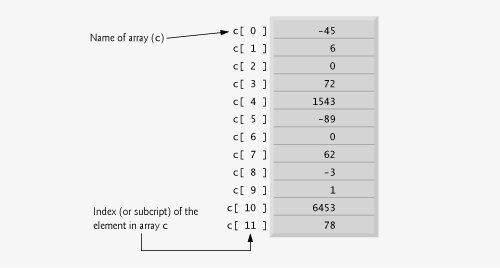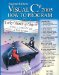# Arrays

An array is a group of variables (called elements) containing values that all have the same type. Recall that types are divided into two categoriesvalue types and reference types. Arrays are reference types. As you will see, what we typically think of as an array is actually a reference to an array instance in memory. The elements of an array can be either value types or reference types (including other arrays, as we will see in Section 8.10). To refer to a particular element in an array, we specify the name of the reference to the array and the position number of the element in the array. The position number of the element is called the element's index.

Figure 8.1 shows a logical representation of an integer array called c. This array contains 12 elements. An application refers to any one of these elements with an array-access expression that includes the name of the array, followed by the index of the particular element in square brackets ([]). The first element in every array has index zero and is sometimes called the zeroth element. Thus, the elements of array c are c[ 0 ], c[ 1 ], c[ 2 ] and so on. The highest index in array c is 11, which is one less than the number of elements in the array, because indices begin at 0. Array names follow the same conventions as other variable names.

Figure 8.1. A 12-element array.An index must be a nonnegative integer and can be an expression. For example, if we assume that variable a is 5 and variable b is 6, then the statement

```c[ a + b ] += 2;
```

adds 2 to array element c[ 11 ]. Note that an indexed array name is an array-access expression. Such expressions can be used on the left side of an assignment to place a new value into an array element. The array index must be a value of type int, uint, long or ulong, or a value of a type that can be implicitly promoted to one of these types.

Let's examine array c in Fig. 8.1 more closely. The name of the array is c. Every array instance knows its own length and provides access to this information with the Length property. For example, the expression c.Length uses array c's Length property to determine the length of the array. Note that the Length property of an array cannot be changed, because it does not provide a set accessor. The array's 12 elements are referred to as c[ 0 ], c[ 1 ], c[ 2 ], ..., c[ 11 ]. It is an error to refer to elements outside of this range, such as c[ -1 ] or c[ 12 ]. The value of c[ 0 ] is -45, the value of c[ 1 ] is 6, the value of c[ 2 ] is 0, the value of c[ 7 ] is 62 and the value of c[ 11 ] is 78. To calculate the sum of the values contained in the first three elements of array c and store the result in variable sum, we would write

```sum = c[ 0 ] + c[ 1 ] + c[ 2 ];
```

To divide the value of c[ 6 ] by 2 and assign the result to the variable x, we would write

```x = c[ 6 ] / 2;
```Visual C# 2005 How to Program (2nd Edition)
ISBN: 0131525239
EAN: 2147483647
Year: 2004
Pages: 600

Similar book on Amazon